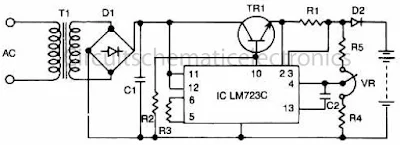# Accu charger with IC LM723C

Circuit of 12 V accu / battery charger with IC LM723C. This Accu charger have input from the first transformer - voltage 220 initially V/110V/240V,  the input voltage lowered approximately 12 VAC, then voltage 12 Volts will be rectified by D1 and then filtered by C1 , then filtered and amplified again by IC LM723 and NPN transistor , output voltage is ready to use to charging the accu / battery.Accu charger with IC LM723C
Part list :
T1 = Transformer , DC 12 V
D1 = Diode bridge
C1 = 470uF /50V
C2 = 1000pF
R1 = 4R7
R2 = 5K
R3 = 3K9
R4 = 7K5
R5 = 8K2
VR = 2K - 5K trim
TR1 = MJ2840
IC = LM723C